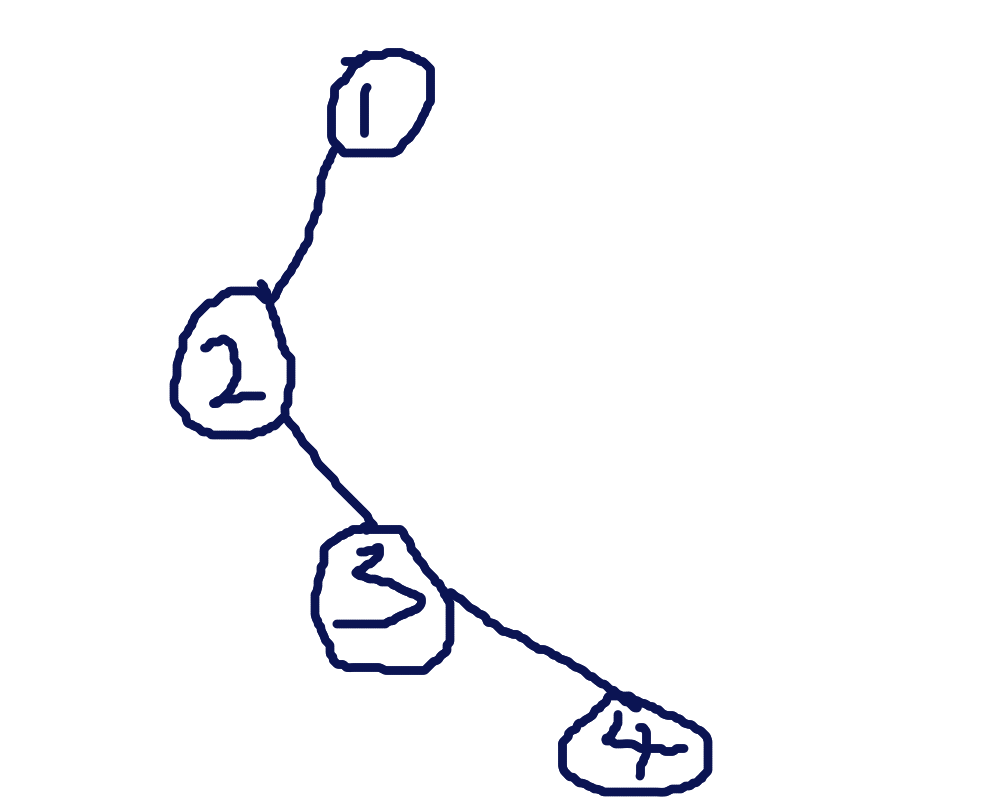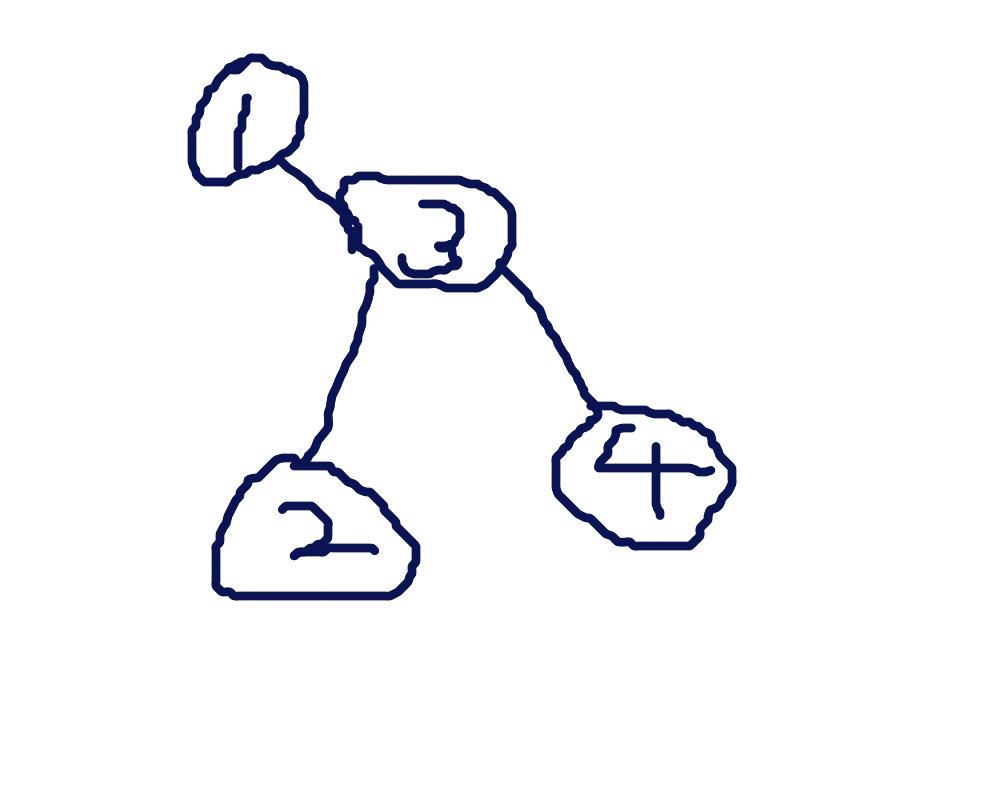#### 吐槽

#define fo(i, a, b) for (int i = (a); i <= (b); ++i)
#define fd(i, a, b) for (int i = (a); i >= (b); --i)
#define N 300005
#define ls(u) t[u].s
#define rs(u) t[u].s


# 概念 (what’s LCT)# LCT 的一些操作

 access(u)

inline void access (int u)
{
for (int las = 0; u; las = u, u = t[u].fa)
splay(u), rs(u) = las, update(u);
}

 toroot(u)

inline void toroot (int u)
{
access(u); splay(u);
std::swap(ls(u), rs(u));
t[rs(u)].rev ^= 1;
}

 findroot(u)

inline int findroot (int u)
{
access(u); splay(u);
while (ls(u)) {pushdown(u); u = ls(u);}
return u;
}

 link(u,v)

inline bool link (int u, int v)
{
toroot(u);
if (findroot(v) == u) return 0;
t[u].fa = v;
return 1;
}

 split(u,v)

inline void split (int u, int v) {toroot(u); access(v); splay(v);}

 cut(u,v)

update: 这里我第一次写的时候 cut 写假了，如果没有保证 cut 合法的情况下，应该如这个代码里面那样写，要注意！！！(模板题代码懒得改了)

inline void cat (int u, int v)
{
split(u, v);
if (findroot(u) == findroot(v) && ls(v) == u && !rs(u))
{
t[u].fa = 0; ls(v) = 0;
update(v);
}
}


# 其它的一些操作

 isroot(u)

inline bool isroot (int u) {int fa = t[u].fa; return ls(fa) == u || rs(fa) == u;}

 rotate(u)

inline void con (int u, int v, int p) {t[v].fa = u; t[u].s[p] = v;}
inline void rotate (int u)
{
int f = t[u].fa, g = t[f].fa, gs = pos(f), fs = pos(u);
con(f, t[u].s[!fs], fs);
if (isroot(f)) con(g, u, gs); else t[u].fa = g;
con(u, f, !fs);
update(f); update(u);
}

 splay(u)

inline void splay (int u)
{
tot = 0;
while (isroot(u)) S[++tot] = u, u = t[u].fa;
S[++tot] = u;
fd (i, tot, 1) pushdown(S[i]);
u = S;
for (; isroot(u); rotate(u))
if (isroot(t[u].fa))
rotate(pos(u) ^ pos(t[u].fa) ? u : t[u].fa);
}


#include<bits/stdc++.h>
#define fo(i, a, b) for (int i = (a); i <= (b); ++i)
#define fd(i, a, b) for (int i = (a); i >= (b); --i)
#define N 300005
#define ls(u) t[u].s
#define rs(u) t[u].s
int data[N], n, root, q, m;
struct tree{
int fa, s, sum;
bool rev;
}t[N];
int S[N], tot;
inline bool isroot (int u) {int fa = t[u].fa; return ls(fa) == u || rs(fa) == u;}
inline bool pos (int u) {return rs(t[u].fa) == u;}
inline void con (int u, int v, int p) {t[v].fa = u; t[u].s[p] = v;}
inline void update (int u) {t[u].sum = t[ls(u)].sum ^ t[rs(u)].sum ^ data[u];}
inline void pushdown (int u)
{
if (t[u].rev)
{
std::swap(ls(u), rs(u));
t[ls(u)].rev ^= 1; t[rs(u)].rev ^= 1;
t[u].rev = 0;
}
}
inline void rotate (int u)
{
int f = t[u].fa, g = t[f].fa, gs = pos(f), fs = pos(u);
con(f, t[u].s[!fs], fs);
if (isroot(f)) con(g, u, gs); else t[u].fa = g;
con(u, f, !fs);
update(f); update(u);
}
inline void splay (int u)
{
tot = 0;
while (isroot(u)) S[++tot] = u, u = t[u].fa;
S[++tot] = u;
fd (i, tot, 1) pushdown(S[i]);
u = S;
for (; isroot(u); rotate(u))
if (isroot(t[u].fa))
rotate(pos(u) ^ pos(t[u].fa) ? u : t[u].fa);
}
inline void access (int u)
{
for (int las = 0; u; las = u, u = t[u].fa)
splay(u), rs(u) = las, update(u);
}
inline void toroot (int u)
{
access(u); splay(u);
std::swap(ls(u), rs(u));
t[rs(u)].rev ^= 1;
}
inline void split (int u, int v) {toroot(u); access(v); splay(v);}
inline int findroot (int u)
{
access(u); splay(u);
while (ls(u)) {pushdown(u); u = ls(u);}
return u;
}
inline bool link (int u, int v)
{
toroot(u);
if (findroot(v) == u) return 0;
t[u].fa = v;
return 1;
}
inline void cat (int u, int v)
{
split(u, v);
if (ls(v) == u)
{
t[u].fa = 0; ls(v) = 0;
update(v);
}
}
inline int query (int u, int v)
{
split(u, v);
return t[v].sum;
}
inline void modify (int u, int val)
{
splay(u); data[u] = val; update(u);
}
int main ()
{
scanf("%d %d", &n, &m);
fo (i, 1, n) scanf("%d", &data[i]);
fo (i, 1, m)
{
int opt, x, y;
scanf("%d %d %d", &opt, &x, &y);
if (!opt) printf("%d\n", query(x, y)); else
if (opt == 1) link(x, y); else
if (opt == 2) cat(x, y); else
modify(x, y);
}
return 0;
}


flashhu 的学习笔记

Candy 学习笔记的第一句

Link, cut, access the chain.

Split your tree, rotate on splay.

update: 附 snakes 新作